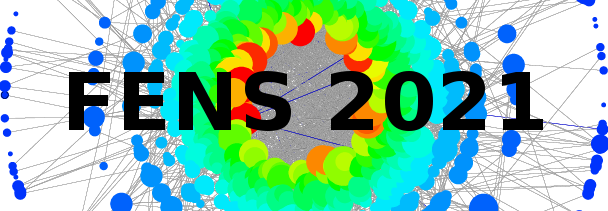#11th Polish Symposium on Physics in Economy and Social Sciences

1-3 July 2021
ONLINE
Europe/Warsaw timezone

## Information Measure for Long Range Correlated Time Series

2 Jul 2021, 17:09
1m
ONLINE

Poster

### Speaker

Pietro Murialdo (Politecnico di Torino)

### Description

We applied the moving average cluster entropy to study long-range correlation, dynamics and heterogeneity of financial time series, and to propose an alternative method to portfolio estimation.

The cluster entropy relies on the Shannon entropy $S(P_i) = - \sum_i P_i \ln P_i$. The probability distribution function of each asset $i$ is obtained intersecting the asset time series $y(t)$ with its moving average series $y_n(t)$. The portion of time series between two consecutive intersections of $y(t)$ and $y_n(t)$ is defined as a cluster. Then, $P(\tau,n)$, which associates clusters of duration $\tau$ to their frequency, is fed into the Shannon entropy to obtain the moving average cluster entropy. We summarize the results into the cluster entropy index $I(n)$, obtained integrating $S(\tau,n)$ over duration $\tau$.

We used high frequency financial time series of important market indexes (NASDAQ, DJIA, S\&P500, ...) of 2018. To study their dynamics, we analyzed twelve consecutive temporal horizon, summing up to a whole year.

We found positive long-range correlation for financial data, similar to fractional stochastic process with Hurst exponent $0.5\leq H\leq 1$.
Moreover, we compared our results to Kullback-Leibler entropy results based on the pricing kernel simulations for different representative agent models.

We show that the cluster entropy of volatility series depends on the individual asset, while the cluster entropy of price series is invariant for different assets. Furthermore, we propose the cluster entropy portfolio. Portfolio weights are obtained from the cluster entropy index $I$. We also integrated horizon dependence. We show the cluster entropy portfolio ability to adjust to market dynamics by varying asset allocation.

### Primary author

Pietro Murialdo (Politecnico di Torino)

### Co-authors

Prof. Anna Carbone (Politecnico di Torino) Prof. Linda Ponta (LIUC - Libera Università Cattaneo)

### Presentation Materials

 FENS2021_Poster_Pietro_Murialdo.pdf Information Measure for Long Range Correlated Time Series Time -- Meeting ID: 729 3406 3812 -- Passcode: FENS11